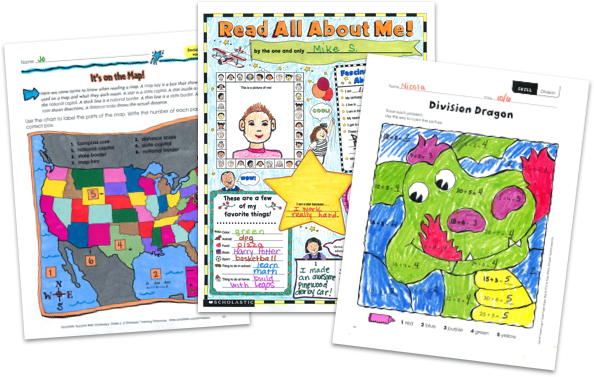MY FILE CABINET# Second Grade Math Worksheets From Scholastic TeachablesScholastic Teachables (formerly Scholastic Printables) has nearly 2,000 grade 2 math worksheets that span many different math skills! Sign up today and get instant online access to 2nd grade math worksheets, as well as math lesson plans, practice pages, and more to cover all different math skills.

Scholastic Teachables (formerly Scholastic Printables) has nearly 2,000 grade 2 math worksheets that span many different math skills! Sign up today and get instant online access to 2nd grade math worksheets, as well as math lesson plans, practice pages, and more to cover all different math skills.

## Scholastic's 2nd Grade Math Worksheets Cover:

• Charts and Graphs
• Estimating
• Fractions
• Geometry
• Glyphs
• Logic and Problem Solving
• Money
• Multiplication
• Number Lines and Plots
• Number Sense
• Patterns
• Place Value
• Real-World Math
• Sequence
• Shapes
• Skip Counting
• Sorting
• Time and Measurement
• Types of Numbers
• Word Problems

## Sample 2nd Grade Math Worksheets

Nearly 2,000 second grade math worksheets from Scholastic Teachables span more than 20 different math topics. Sign up and you'll also get access to Scholastic's more than 30,000 award-winning printable resources for every grade and subject!

Here's a sample of worksheets for you to try in your class—FREE with a 30-day trial or subscription.

## Scholastic's 2nd Grade Math Worksheets Cover:

• Charts and Graphs
• Estimating
• Fractions
• Geometry
• Glyphs
• Logic and Problem Solving
• Money
• Multiplication
• Number Lines and Plots
• Number Sense
• Patterns
• Place Value
• Real-World Math
• Sequence
• Shapes
• Skip Counting
• Sorting
• Time and Measurement
• Types of Numbers
• Word Problems
• Charts and Graphs
• Estimating
• Fractions
• Geometry
• Glyphs
• Logic and Problem Solving
• Money
• Multiplication
• Number Lines and Plots
• Number Sense
• Patterns
• Place Value
• Real-World Math
• Sequence
• Shapes
• Skip Counting
• Sorting
• Time and Measurement
• Types of Numbers
• Word Problems

## Sample 2nd Grade Math Worksheets

Nearly 2,000 second grade math worksheets from Scholastic Teachables span more than 20 different math topics. Sign up and you'll also get access to Scholastic's more than 30,000 award-winning printable resources for every grade and subject!

Here's a sample of worksheets for you to try in your class—FREE with a 30-day trial or subscription.## Additional Alphabet Worksheets (Subscription Required)

Try FREE for 30 days!

If you are not 100% satisfied cancel during the FREE trial and owe nothing. If you are not 100% satisfied cancel during the FREE trial and owe nothing.